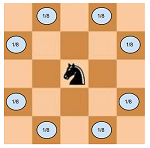# Knight on a Chess Board

962.0K Views

Given the size of the chess board and initial position of the knight, what is the probability that after k moves the knight will be inside the chess board.

Note:-
1) The knight makes its all 8 possible moves with equal probability.
2) Once the knight is outside the chess board it cannot come back inside.This challenge is originally from a blog post of crazyforcode.com published under the CC BY-NC-ND 2.5 IN licence.

After removing mirror images, there are six unique positions on the board: (1,1), (1,2), (1,3), (2,2), (2,3), (3,3).  The knight starts at (3,3).

From each position, there is a fixed probability of moving to each of the other five positions.  The probability of moving off the board is 1 minus the sum of the probabilities of moving to other board positions.

``` .      -------- starting position --------
.      (1,1) (1,2) (1,3) (2,2) (2,3) (3,3)
(1,1)                          .250
(1,2)              .250  .250        1.000
(1,3)        .125              .250
(2,2)        .125              .250
(2,3)  .250        .250  .250
(3,3)        .125
```

A quick examination reveals that positions (1,3) and (2,2) are equivalent–they each have the same probability for the following square.  So we can collapse them into a single state we’ll label as (*,*), giving the following five position table.

``` .      ----- starting position -----
.      (1,1) (1,2) (*,*) (2,3) (3,3)
(1,1)                    .250
(1,2)              .250        1.000
(*.*)        .250        .500
(2,3)  .250        .250
(3,3)        .125
```

The probability for the knight to be in each of the five positions at the end of k moves is the sum of the product of probability of the knight moving to that position from another position and the probability of having been in that position at the end of k-1 moves.  At zero moves, the probability of being in position (3,3) is 1 and the probability of being elsewhere on the board is 0.  After each move, the probability of the knight having moved off the board is 1 minus the sum of the probabilities of it being in each position.

``` k  (1,1)  (1,2)  (*,*)  (2,3)  (3,3)   Off board
0                              1.00000
1         1.00000
2                0.25000       0.12500  0.62500
3         0.18750       0.06250         0.75000
4  0.01563       0.07813       0.02344  0.88281
5         0.04297       0.02344         0.93359
6  0.00586       0.02246       0.00537  0.96631
7         0.01099       0.00708         0.98193
8  0.00177       0.00629       0.00137  0.99057
9         0.00294       0.00201         0.99504
10 0.00050       0.00174       0.00037  0.99739
```

ALGORITHM: